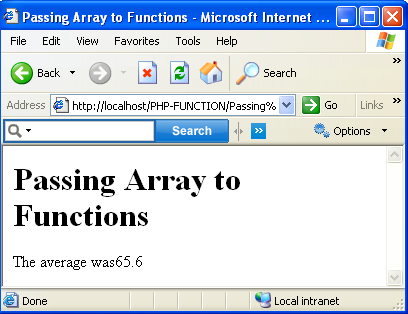# PHP Programing language

How Can Passing Arrays to Functions
 Previous Home Next
You can also pass arrays to functions as easily as simple data items like string or numbers. For example , you might want a function that averages a set of students' test scores and displays that average, called averager. And you might want to pass an array to the averager function Here the look like the array of student test score, and how to might pass the \$scores array to the averager function:

```<?php
\$score= array (66,75,78,55,54);
.
averager(\$scores);
.
?>
```

### you can determine the average value of the array elements and display:

```<html>
<title>Passing Array to Functions </title>
<body>
<h1>Passing Array to Functions </h1>
<?php
\$scores=array(66,75,78,55,54);
averager(\$scores);
function averager(\$array)
{
\$total=0;
foreach (\$array as \$value)
{
\$total += \$value;
}
if(count(\$array)>0)
{
echo "The average was", \$total/count(\$array);
}
else
{
echo "No element to average!";
}
}
?>
</body>
</html>
```

Output:Previous Home Next